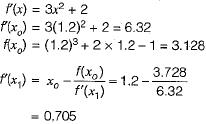Courses

# Probability (Advance Level) - 1

## 15 Questions MCQ Test Question Bank for GATE Computer Science Engineering | Probability (Advance Level) - 1

Description
This mock test of Probability (Advance Level) - 1 for GATE helps you for every GATE entrance exam. This contains 15 Multiple Choice Questions for GATE Probability (Advance Level) - 1 (mcq) to study with solutions a complete question bank. The solved questions answers in this Probability (Advance Level) - 1 quiz give you a good mix of easy questions and tough questions. GATE students definitely take this Probability (Advance Level) - 1 exercise for a better result in the exam. You can find other Probability (Advance Level) - 1 extra questions, long questions & short questions for GATE on EduRev as well by searching above.
QUESTION: 1

### A die is rolled three times. The probability that exactly one odd number turns up among the three outcomes is

Solution:

Probability of getting an odd number in rolling of a die = 3/6 = 1/2.
Now using binomial distribution
P(Exactly one odd number among three outcomes)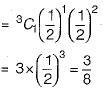QUESTION: 2

### Seven (distinct) car accidents occurred in a week. What is the probability that they all occurred on the same day?

Solution:

Sample space = 77
All accidents on the same day = 7 ways (all on Monday, all on Tuesday...)
So, required probability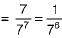QUESTION: 3

### Let X and Y be two exponentially distributed and independent random variables with mean α and β, respectively. If Z= min (X, Y), then the mean of Z is given by

Solution: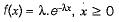X and Y are two independent exponentially distributed random variables. Let λ1 and λ2 parameters of X and Y respectively.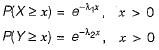Given, Z = min (X, Y)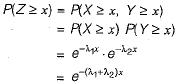Since mean of exponential distribution = 1/Parameter
So,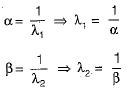∴ Z is random variable with parameter
Mean of Z =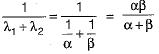QUESTION: 4

A bag contains 10 blue marbles, 20 green marbles and 30 red marbles. A marble is drawn from the bag, its colour recorded and it is put back in the bag. This process is repeated 3 times. The probability that no two of the marbles drawn have the same colour is

Solution:

The given condition corresponds to sampling with replacement and with order.
No 2 marbles have the same color i.e. Drawn 3 different marble.
So total number of ways for picking 3 different marbles = 3! = 6.
Probability of getting blue, green, red in order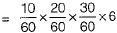[Since 6 ways to get the marbles]
= 1/6

QUESTION: 5

In a certain town, the probability that it will rain in the afternoon is known to be 0.6. Moreover, meteorological data indicates that if the temperature at noon is less than or equal to 250C, the probability that it will rain in the afternoon is 0.4. The temperature at noon is equally likely to be above 250C, or at/below 250C.
What is the probability that it will rain in the afternoon on a day when the temperature at noon is above 250C?

Solution:

Let RA : Rain in the afternoon
T > 25 : Temperature more than 250C
Let the desired probability = P(RAIT ≤ 25) = x
The tree diagram forthis problem is given below: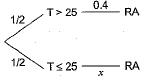Given P(RA) = 0.6
by rule of total probability P(RA)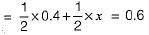⇒ x = 0.8

QUESTION: 6

Suppose a fair six-sided die is rolled once, if the value on the die is 1, 2 or 3 then die is rolled a second time. What is the probability that the sum total of values that turn up is at least 6?

Solution:

If first throw is 1, 2 or 3 then sample space is only 18 possible ordered pairs. Out of this only (1, 5 ), (1, 6 ), (2, 4), (2, 5), (2, 6), (3, 3), (3, 4), (3, 5) and (3,6) i e. 9 out of 18 ordered pairs gives a Sum ≥ 6.
If first throw is 4, 5 or 6 then second throw is not made and therefore the only way Sum ≥ 6 is if the throw was 6. Which is one out of 3 possible. So the tree diagram becomes as follows: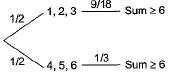From above diagram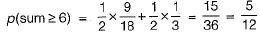QUESTION: 7

Suppose p is the number of cars per minute passing through a certain road junction between 5 PM and p has Poisson distribution with mean 3. What is the probability of observing fewer than 3 cars during any given minute in this interval?

Solution: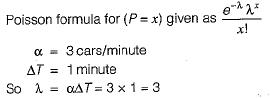Probability of observing fewer than 3 cars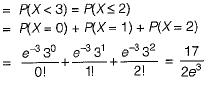QUESTION: 8

A fair coin is tossed till a head appears for the first time. The probability that the number of required tosses is odd, is

Solution:

P(number of tosses is odd) = P(number of tosses is 1, 3, 5, 7 ...)
P(number of toss is 1) = P(Head in first toss = 1/2
P(number of toss is 3) = P(tail in first toss, tail in second toss and head in third toss)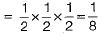P(number of toss is 5) = P(T, T, T, T, H)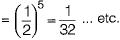So P(number of tosses is odd)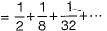Sum of infinite geometric series with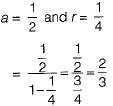QUESTION: 9

A continuous random variable Xhas a probability density function f(x) = e-x, 0 < x <∝. Then P{X> 1} is

Solution: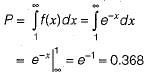QUESTION: 10

A fair coin is tossed n times. The probability that the difference between the number of heads and tails is ( n - 3) is

Solution: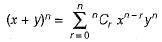when n = probability of occurrence of head
y = probability of occurrence of tail
Let number of head is P
Number of tail is q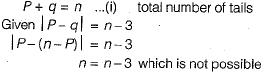Here required probability is zero.

QUESTION: 11

Two players, A and B, alternately keep rolling a fair dice. The person to get a six first wins the game. Given that player A starts the game, the probability that A wins the game is

Solution:

P(A wins) = p(6 in first throw by A) + p(A not 6, B not 6, A 6) + ...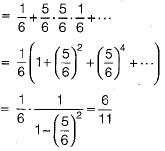QUESTION: 12

Equation ex - 1 = 0 is required to be solved using Newton's method with an initial guess x0 = -1. Then, after one step of Newton’s method, estimate x1 of the solution will be given by

Solution: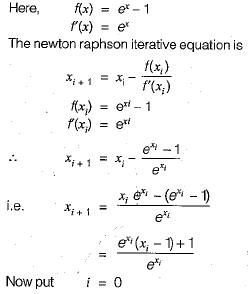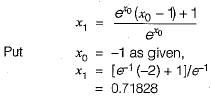QUESTION: 13

Let x2 - 117 = 0. The iterative steps for the solution using Newton-Raphson’s method is given by

Solution: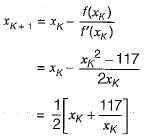QUESTION: 14

Solution of the variables x1 and x2 for the following equations is to be obtained by employing the Newton-Raphson iterative method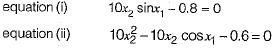Assuming the initial values x1 = 0.0 and x2 = 1.0, the Jacobian matrix is

Solution: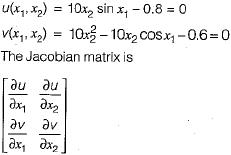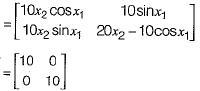QUESTION: 15

When the Newton-Raphson method is applied to solve the equation f(x) = x3 + 2x - 1 = 0, the solution at the end of the first iteration with the initial guess value as x0 = 1.2 is

Solution: